# Electronics and Communication Engineering - Microwave Communication

### Exercise :: Microwave Communication - Section 8

6.

In rectangular waveguides the ratio of width to height is about

 A. 0.5 B. 1 C. 2 D. 4

Explanation:

No answer description available for this question. Let us discuss.

7.

If wavelength in free space is λ0 wavelength in insulator λ, μr is relative permittivity or insulator and ∈r is relative permittivity or insulator, then

 A.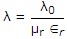B.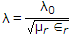C.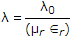D.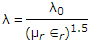Explanation:

No answer description available for this question. Let us discuss.

8.

In the given figure the circuits for input impedance of a microwave device with output ports match terminated. The device is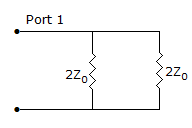A. E plane tee B. H plane tee C. magic tee D. hybrid ring

Explanation:

No answer description available for this question. Let us discuss.

9.

Which of these statements is correct?

 A. Ridge guide is essentially a form of capacitively loaded guide. B. Ridge guide is essentially a form of inductively loaded guide. C. Ridge guide is essentially a form of resistively loaded guide. D. Ridge guide has higher power handling capacity than rectangular waveguide.

Explanation:

No answer description available for this question. Let us discuss.

10.

A rectangular waveguide has width a. Then at the cutoff frequency (with corresponding wave length λc)

 A.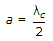B. a = λc C.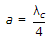D. a = 2λc